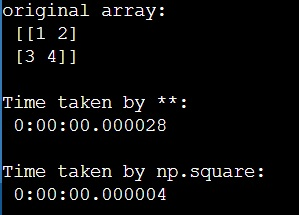# numpy.square() Method vs ** Operator

Learn about the numpy.square() method, and ** Operator. What is the difference between them?
Submitted by Pranit Sharma, on March 22, 2023

## numpy.square() vs **

The numpy.square() returns the element-wise square of the input. On the other hand, the ** operator is another method to find the square of a given number. While applying this method, the exponent operator returns the exponential power resulting in the square of the number. Here, a**b refers to "a to the power of b".

Both of the methods give the same results. Generally, the standard pythonic a**b is slightly slower than the numpy.square(), and also, numpy functions are often more flexible and precise. If we do calculations that need to be very accurate, we should use numpy and probably even use other datatypes like floats.

Let us see the performance timings of both methods,

## Python code to demonstrate the difference between numpy.square() method and ** Operator

```# Import numpy
import numpy as np

# Import datetime
from datetime import datetime

# Creating a numpy array
arr = np.array([[1,2],[3,4]])

# Display original array
print("original array:\n",arr,"\n")

# using **
start = datetime.now()
(arr ** 2)
stop = datetime.now()

# Display result
print("Time taken by **:\n",stop-start,"\n")

# using np.square
start = datetime.now()
(np.square(arr))
stop = datetime.now()

# Display result
print("Time taken by np.square:\n",stop-start,"\n")
```

### Output